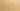# VariablesHoldExpressions

Misconception:

For example, `int a = v/2` will store the expression `v/2` in the variable `a`.Incorrect

= stores an expression in a variable

Correct

= evaluates an expression and stores its value in a variable

## CorrectionHere is what's right.

First, the expression is evaluated. Then the assignment operator (`=`) stores the resulting value in the variable.

Variables hold values. In Java, an expression is not a value. Thus, variables cannot store expressions. However, an expression can be evaluated, producing a value. That value can then be stored in the variable.

Note that once a value is produced, there is no more connection between that value and the expression that produced it.

## OriginWhere could this misconception come from?

This misconception may at least partially be due to students’ familiarity with algebra, where the right hand side of an equation is not evaluated and stored in a variable on the left.

While chances are low, it could be that a student got exposed to “pass-by-name” beforehand.

## SymptomsHow do you know your students might have this misconception?

A student holding this misconception may put the expression (not its value) into the box of a memory snapshot diagram. Alternatively, the student may draw an arrow from the box to a heap object holding that expression.

## ValueHow can you build on this misconception?

This misconception can be very valuable when introducing functional programming, where functions (including anonymous functions, lambdas) are treated as normal values.

If a student (wrongfully) thinks that the following statement stores the unevaluated expression `a + 1` in variable `inc`

``inc = a + 1;``

…then when introducing lambdas one can explain that the following statement does do that:

``inc = a -> a + 1;``

Java